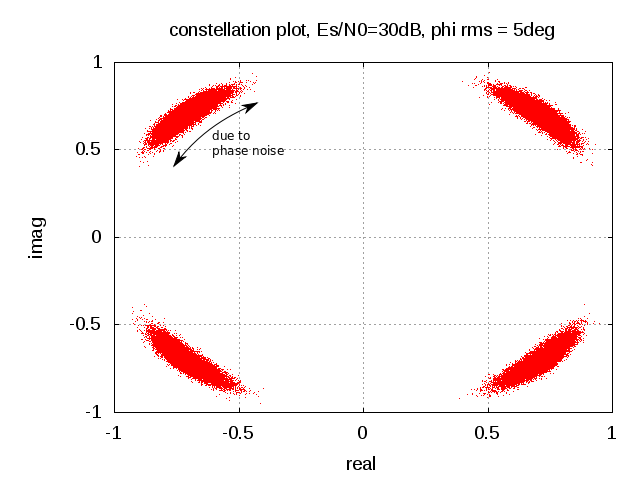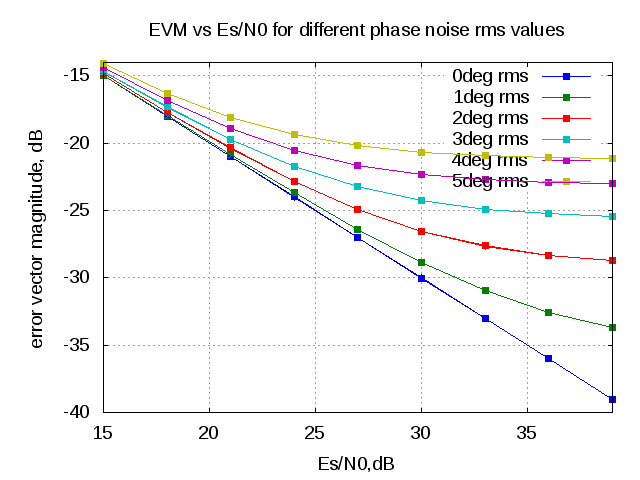- DSP log - http://www.dsplog.com -

EVM with phase noise

Posted By Krishna Sankar On July 9, 2012 @ 6:30 am In Analog | 9 Comments

The previous post on phase noise discussed about finding the root mean square phase noise for a given phase noise profile . In this post let us discuss about the impact of phase noise on the error vector magnitude (evm) of a transmit symbol.

Error Vector Magnitude due to constant phase offset

Consider a system model introducing a constant phase offset and thermal noise  as shown in the figure.Figure : System model with phase noise and thermal noise

,

where

is the phase distortion in radians,

is the transmit symbol and

is the contribution due to thermal noise

Expanding into real and imaginary components,

.

Representing in matrix algebra,

The power of the error vector is,

.

Finding the average error over many realizations,

.

The individual terms can be simplified as,

i)

as .

ii)

as and are uncorrelated.

iii) .

iv) , the variance of the noise.

Applying (i), (ii) , (iii) and (iv), the error term simplifies to

.

EVM due to random phase offset

The above equation derives the evm when the system is affected by a constant phase offset .   Assume that the phase is Gaussian distributed with zero mean and variance  radians^2 having a probability density function as,

.

The conditional error power for a given phase  is,

.

Computing the average over all realization of phase,

.

The integral term is,

(Note : proof will be discussed in another post)

Then the error vector power is,

and the error vector magnitude is,

Using Taylor series, and assuming that the  is small,

,Figure : Example constellation plot (Es/N0=30dB, =5 degrees)

Matlab/Octave example

Attached script computes the evm of a QPSK modulated symbol versus Es/N0 for different values of rms phase noise.

% Script for simulating the error vector magnitude (evm) of a QPSK
% modulated symbol affected by phase noise and thermal noise
% ----------------------------------------------------------

clear;close all;
N = 10^5 % number of bits or symbols

Es_N0_dB = [15:3:40]; % multiple Eb/N0 values
phi_rms_deg_vec = [0:1:5];

for ii = 1:length(Es_N0_dB)
for jj = 1:length(phi_rms_deg_vec)
% Transmitter
ip_re = rand(1,N)>0.5; % generating 0,1 with equal probability
ip_im = rand(1,N)>0.5; % generating 0,1 with equal probability
s = 1/sqrt(2)*(2*ip_re-1 + j*(2*ip_im-1)); % QPSK modulation

n = 1/sqrt(2)*[randn(1,N) + j*randn(1,N)]; % thermal noise
phi = phi_rms_deg_vec(jj)*(pi/180)*randn(1,N); % phase noise

y = s.*exp(j*phi) + 10^(-Es_N0_dB(ii)/20)*n;

% error vector
error_vec = (y-s);

evm(ii,jj)  = error_vec*error_vec';
theory_evm(ii,jj) =  10^(-Es_N0_dB(ii)/10) + 2 - 2*exp(-(phi_rms_deg_vec(jj)*pi/180).^2/2);
end
end

figure;
plot(Es_N0_dB,10*log10((evm/N)),'s-');
hold on;grid on
plot(Es_N0_dB,10*log10(theory_evm),'+-');
xlabel('Es/N0,dB');
ylabel('error vector magnitude, dB');
title('EVM vs Es/N0 for different phase noise rms values');
legend('0deg rms','1deg rms','2deg rms','3deg rms','4deg rms','5deg rms')Figure : EVM vs Es/N0 for different values of rms phase noise

Summary

As a quick rule of thumb, for a system  with rms phase noise of 1 degree, the evm due to phase noise alone is -35.16dB and rises by 6dB per octave (or 20dB per decade).

The phase noise profile used in this simulations assumes a Gaussian distributed flat spectrum, which is not the case in typical phase noise profiles. The EVM with a classical phase noise profile will be discussed in another post.

References

 Georgiadis, A.; , “Gain, phase imbalance, and phase noise effects on error vector magnitude,” Vehicular Technology, IEEE Transactions on , vol.53, no.2, pp. 443- 449, March 2004
doi: 10.1109/TVT.2004.823477
URL: http://ieeexplore.ieee.org/stamp/stamp.jsp?tp=&arnumber=1275708&isnumber=28551